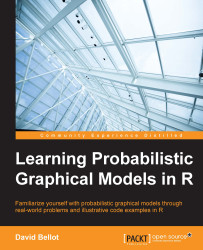•#### Learning Probabilistic Graphical Models in R#### Overview of this book

Probabilistic graphical models (PGM, also known as graphical models) are a marriage between probability theory and graph theory. Generally, PGMs use a graph-based representation. Two branches of graphical representations of distributions are commonly used, namely Bayesian networks and Markov networks. R has many packages to implement graphical models. We’ll start by showing you how to transform a classical statistical model into a modern PGM and then look at how to do exact inference in graphical models. Proceeding, we’ll introduce you to many modern R packages that will help you to perform inference on the models. We will then run a Bayesian linear regression and you’ll see the advantage of going probabilistic when you want to do prediction. Next, you’ll master using R packages and implementing its techniques. Finally, you’ll be presented with machine learning applications that have a direct impact in many fields. Here, we’ll cover clustering and the discovery of hidden information in big data, as well as two important methods, PCA and ICA, to reduce the size of big problems.
Learning Probabilistic Graphical Models in RCreditswww.PacktPub.comPrefaceFree Chapter
Probabilistic ReasoningExact InferenceLearning ParametersBayesian Modeling – Basic ModelsApproximate InferenceBayesian Modeling – Linear ModelsProbabilistic Mixture ModelsAppendixIndex## Chapter 6. Bayesian Modeling – Linear Models

A linear regression model aims at explaining the behavior of one variable with another one, or several others, and by so doing, the assumption is that the relationship between the variables is linear. In general, the expectation of the target variable, the one you need to explain, is an affine transform of several other variables.

Linear models are presumably the most used statistical models, mainly because of their simplicity and the fact they have been studied for decades, leading to all possible extensions and analysis one can imagine. Basically all statistical packages, languages, or software implement linear regression models.

The idea of the model is really simple: a variable y is to be explained by several other variables xi by assuming a linear combination of x's—that is, a weighted sum of x's.

This model appeared in the 18th century in the work of Roger Joseph Boscovich. Then again, his method has been used by Pierre-Simon de Laplace, Adrien...